# 7 Types of Activation Functions in Neural Network

• Dinesh Kumawat
• Aug 22, 2019
• Deep Learning
• Updated on: Jan 16, 2021Activation functions are the most crucial part of any neural network in deep learning. In deep learning, very complicated tasks are image classification, language transformation, object detection, etc which are needed to address with the help of neural networks and activation function. So, without it, these tasks are extremely complex to handle.

In the nutshell, a neural network is a very potent technique in machine learning that basically imitates how a brain understands, how? The brain receives the stimuli, as input, from the environment, processes it and then produces the output accordingly.

## Introduction

The neural network activation functions, in general, are the most significant component of Deep Learning, they are fundamentally used for determining the output of deep learning models, its accuracy, and performance efficiency of the training model that can design or divide a huge scale neural network.

Activation functions have left considerable effects on the ability of neural networks to converge and convergence speed, don’t you want to how? Let’s continue with an introduction to the activation function, types of activation functions & their importance and limitations through this blog.

## What is the activation function?

Activation function defines the output of input or set of inputs or in other terms defines node of the output of node that is given in inputs. They basically decide to deactivate neurons or activate them to get the desired output. It also performs a nonlinear transformation on the input to get better results on a complex neural network.

Activation function also helps to normalize the output of any input in the range between 1 to -1. Activation function must be efficient and it should reduce the computation time because the neural network sometimes trained on millions of data points.

Activation function basically decides in any neural network that given input or receiving information is relevant or it is irrelevant. Let's take an example to understand better what is a neuron and how activation function bounds the output value to some limit.

The neuron is basically is a weighted average of input, then this sum is passed through an activation function to get an output.

Y = ∑ (weights*input + bias)

Here Y can be anything for a neuron between range -infinity to +infinity. So, we have to bound our output to get the desired prediction or generalized results.

Y = Activation function(∑ (weights*input + bias))

So, we pass that neuron to activation function to bound output values.

## Why do we need Activation Functions?

Without activation function, weight and bias would only have a linear transformation, or neural network is just a linear regression model, a linear equation is polynomial of one degree only which is simple to solve but limited in terms of ability to solve complex problems or higher degree polynomials.

But opposite to that, the addition of activation function to neural network executes the non-linear transformation to input and make it capable to solve complex problems such as language translations and image classifications.

In addition to that, Activation functions are differentiable due to which they can easily implement back propagations, optimized strategy while performing backpropagations to measure gradient loss functions in the neural networks.

## Types of Activation Functions

The most famous activation functions are given below,

1. Binary step

2. Linear

3. ReLU

4. LeakyReLU

5. Sigmoid

6. Tanh

7. Softmax

### 1. Binary Step Activation Function

This activation function very basic and it comes to mind every time if we try to bound output. It is basically a threshold base classifier, in this, we decide some threshold value to decide output that neuron should be activated or deactivated.

f(x) = 1 if x > 0  else 0 if x < 0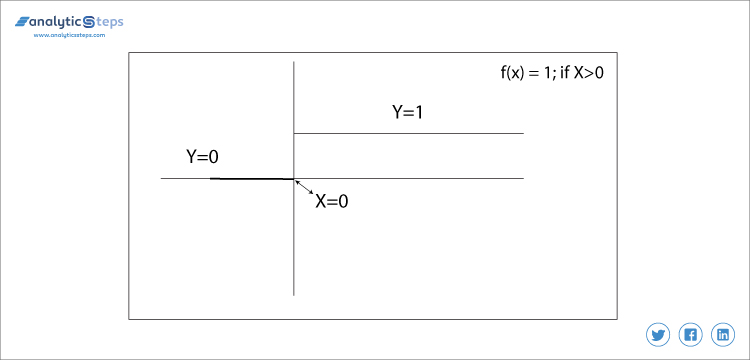Binary step function

In this, we decide the threshold value to 0. It is very simple and useful to classify binary problems or classifier.

### 2. Linear Activation Function

It is a simple straight line activation function where our function is directly proportional to the weighted sum of neurons or input. Linear activation functions are better in giving a wide range of activations and a line of a positive slope may increase the firing rate as the input rate increases.

In binary, either a neuron is firing or not. If you know gradient descent in deep learning then you would notice that in this function derivative is constant.

Y = mZ

Where derivative with respect to Z is constant m. The meaning gradient is also constant and it has nothing to do with Z. In this, if the changes made in backpropagation will be constant and not dependent on Z so this will not be good for learning.

In this, our second layer is the output of a linear function of previous layers input. Wait a minute, what have we learned in this that if we compare our all the layers and remove all the layers except the first and last then also we can only get an output which is a linear function of the first layer.

### 3. ReLU( Rectified Linear unit) Activation function

Rectified linear unit or ReLU is most widely used activation function right now which ranges from 0 to infinity, All the negative values are converted into zero, and this conversion rate is so fast that neither it can map nor fit into data properly which creates a problem, but where there is a problem there is a solution.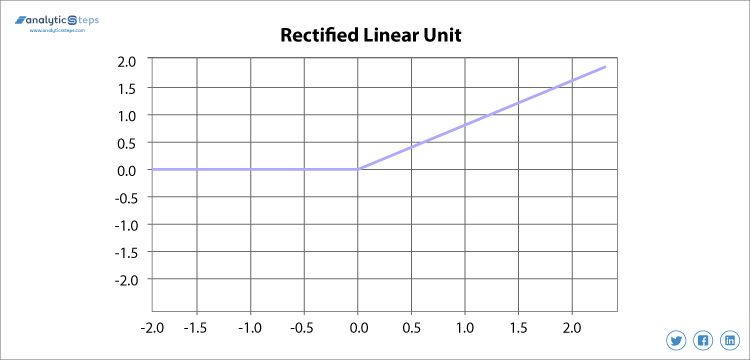Rectified Linear Unit activation function

We use Leaky ReLU function instead of ReLU to avoid this unfitting, in Leaky ReLU range is expanded which enhances the performance.

### 4. Leaky ReLU Activation Function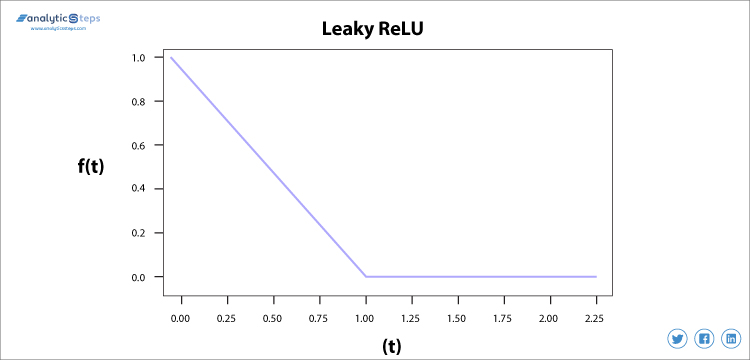Leaky ReLU Activation Function

We needed the Leaky ReLU activation function to solve the ‘Dying ReLU’ problem, as discussed in ReLU, we observe that all the negative input values turn into zero very quickly and in the case of Leaky ReLU we do not make all negative inputs to zero but to a value near to zero which solves the major issue of ReLU activation function.

### 5. Sigmoid Activation Function

The sigmoid activation function is used mostly as it does its task with great efficiency, it basically is a probabilistic approach towards decision making and ranges in between 0 to 1, so when we have to make a decision or to predict an output we use this activation function because of the range is the minimum, therefore, prediction would be more accurate.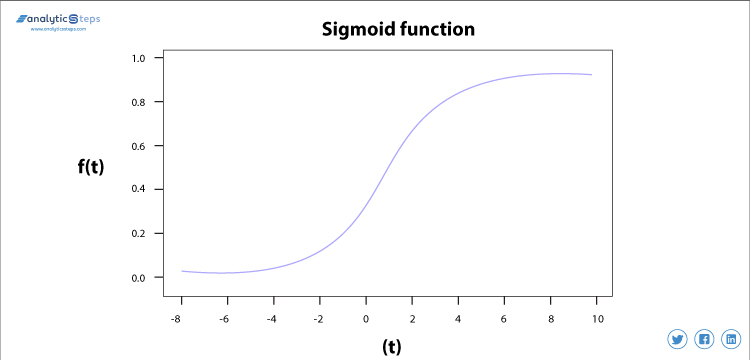Sigmoid Activation function

The equation for the sigmoid function is

f(x) = 1/(1+e(-x) )

The sigmoid function causes a problem mainly termed as vanishing gradient problem which occurs because we convert large input in between the range of 0 to 1 and therefore their derivatives become much smaller which does not give satisfactory output. To solve this problem another activation function such as ReLU is used where we do not have a small derivative problem.

### 6. Hyperbolic Tangent Activation Function(Tanh)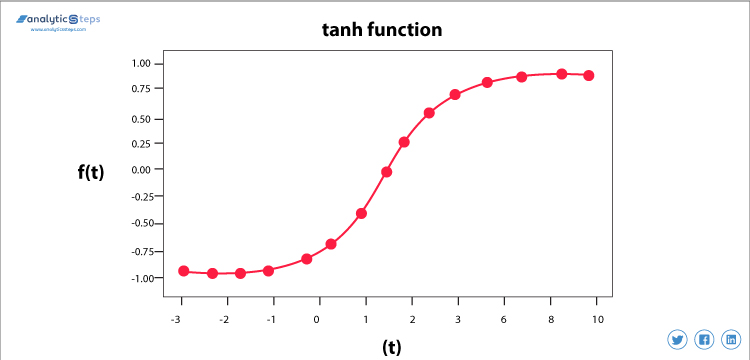Tanh Activation function

This activation function is slightly better than the sigmoid function, like the sigmoid function it is also used to predict or to differentiate between two classes but it maps the negative input into negative quantity only and ranges in between -1 to  1.

### 7. Softmax Activation Function

Softmax is used mainly at the last layer i.e output layer for decision making the same as sigmoid activation works, the softmax basically gives value to the input variable according to their weight and the sum of these weights is eventually one.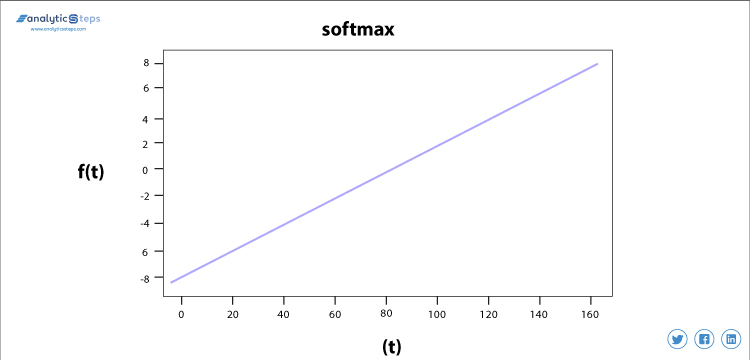Softmax on Binary Classification

For Binary classification, both sigmoid, as well as softmax, are equally approachable but in case of multi-class classification problem we generally use softmax and cross-entropy along with it.

## Conclusion

The activation functions are those significant functions that perform a non-linear transformation to the input and making it proficient to understand and executes more complex tasks. We have discussed 7 majorly used activation functions with their limitation (if any), these activation functions are used for the same purpose but in different conditions.

Now you may finally know the importance of activation functions in neural networks. For more blogs in Analytics and new technologies do read Analytics Steps.

0%

•#### rajatktiwari1997

May 22, 2020

The importance of activation function has never been explained more vividly than this .

•